Abstract: 数字图像处理：第21天
Keywords: 离散傅里叶变换

## 离散傅里叶变换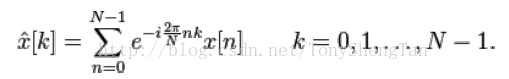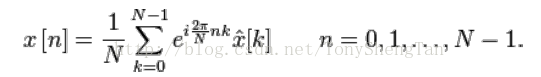## 数学推导

$$x[n]=x(k\times T),k=0,1,2,3,\dots N-1$$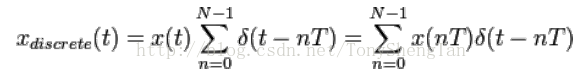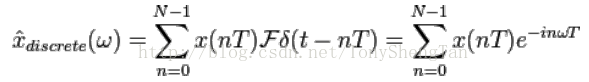$-w>-\frac{2\times\pi}{2\times T}$ ，所以离散序列对应的频率宽度为 $\frac{2\times\pi}{T}$ 。因为原序列的长度为L所以其最小频率间隔为 $\frac{2\times\pi}{L}$ 。那么频率域的离散序列长度为 $\frac{\frac{2\times\pi}{T}}{\frac{2\times\pi}{L}}=\frac{L}{T}=N$ ，与原序列对应，顾频率域的采样间隔为 $\frac{2\pi}{N}$ 。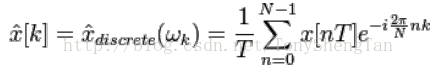## 性质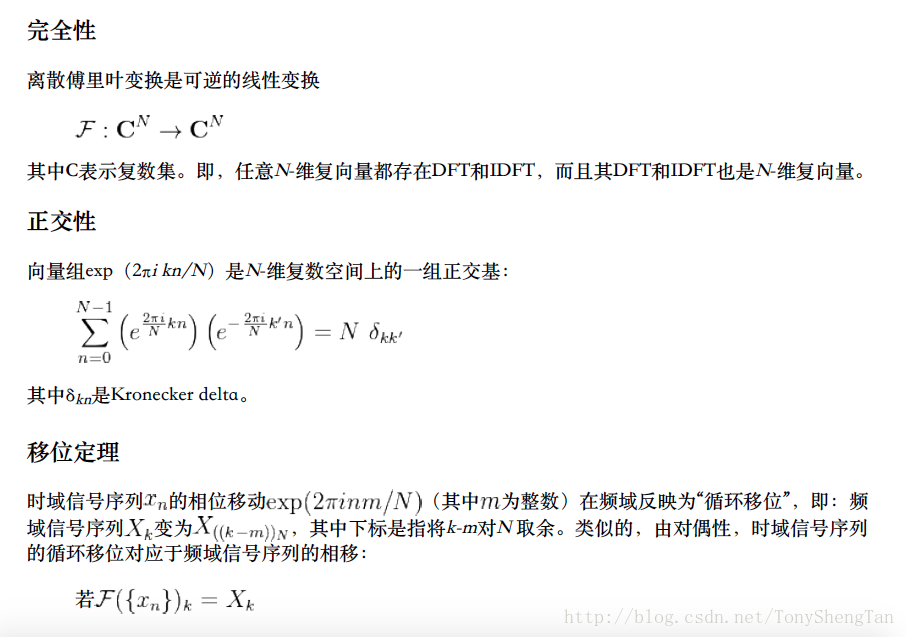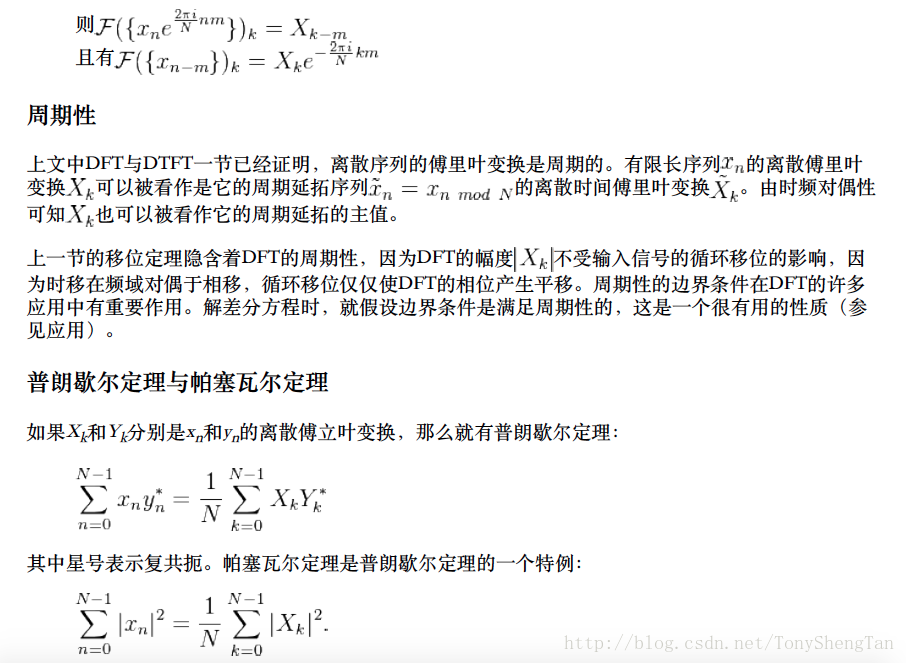## 总结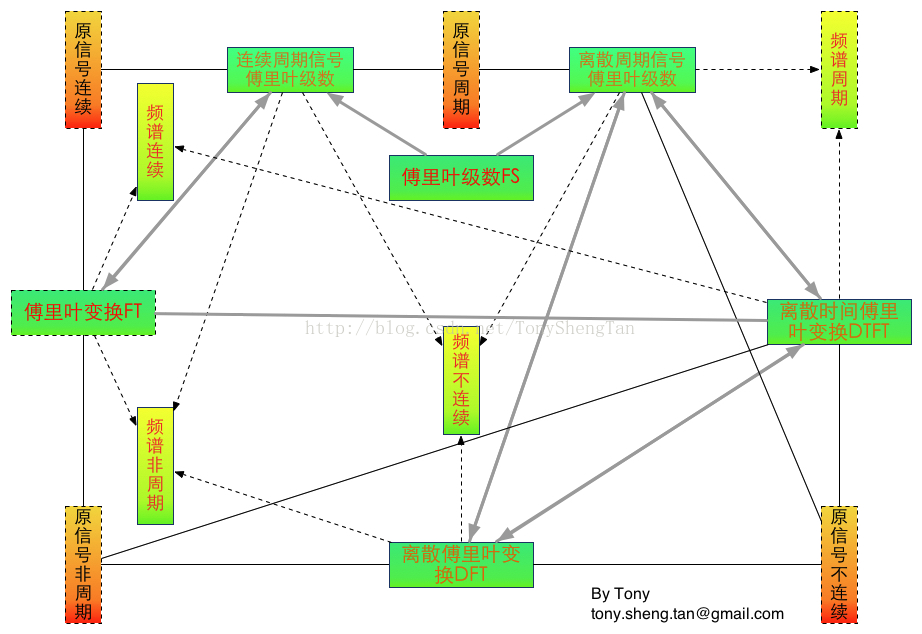0%